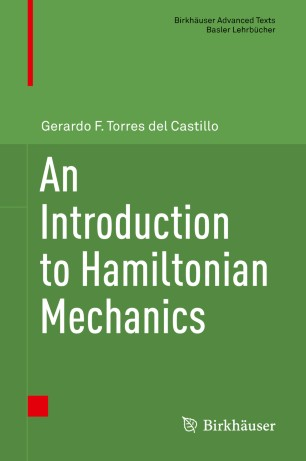# An Introduction to Hamiltonian Mechanics

• Gerardo F. Torres del CastilloTextbook

Part of the Birkhäuser Advanced Texts Basler Lehrbücher book series (BAT)

1. Front Matter
Pages i-x
2. Gerardo F. Torres del Castillo
Pages 1-41
3. Gerardo F. Torres del Castillo
Pages 43-80
4. Gerardo F. Torres del Castillo
Pages 81-101
5. Gerardo F. Torres del Castillo
Pages 103-141
6. Gerardo F. Torres del Castillo
Pages 143-228
7. Gerardo F. Torres del Castillo
Pages 229-279
8. Back Matter
Pages 281-366

### Introduction

This textbook examines the Hamiltonian formulation in classical mechanics with the basic mathematical tools of multivariate calculus. It explores topics like variational symmetries, canonoid transformations, and geometrical optics that are usually omitted from an introductory classical mechanics course. For students with only a basic knowledge of mathematics and physics, this book makes those results accessible through worked-out examples and well-chosen exercises.

For readers not familiar with Lagrange equations, the first chapters are devoted to the Lagrangian formalism and its applications. Later sections discuss canonical transformations, the Hamilton–Jacobi equation, and the Liouville Theorem on solutions of the Hamilton–Jacobi equation.

Graduate and advanced undergraduate students in physics or mathematics who are interested in mechanics and applied math will benefit from this treatment of analytical mechanics. The text assumes the basics of classical mechanics, as well as linear algebra, differential calculus, elementary differential equations and analytic geometry. Designed for self-study, this book includes detailed examples and exercises with complete solutions, although it can also serve as a class text.

### Keywords

inertia tensor Poisson bracket Hamiltonian mechanics canonical transformations rigid bodies Liouville Theorem Hamilton–Jacobi formalism canonoid transformations Lagrangian formalism Hamilton–Jacobi equation

#### Authors and affiliations

• Gerardo F. Torres del Castillo
• 1
1. 1.Instituto de Ciencias, BUAPPueblaMexico